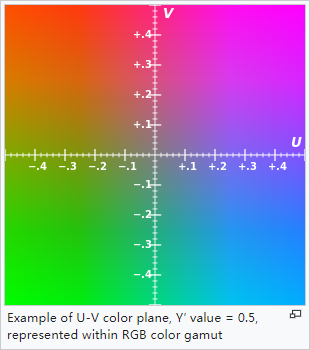YUV is a color coding system, usually used as part of the color image pipeline. It encodes color images or videos in consideration of human perception, thereby allowing the bandwidth of chrominance components to be reduced. Therefore, compared to using "direct" RGB representation, transmission errors or compression artifacts can usually be masked more effectively by human perception.## YUV to RGB Conversion

B = 1.164(Y - 16)  + 2.018(U - 128)

G = 1.164(Y - 16) - 0.813(V - 128) - 0.391(U - 128)

R = 1.164(Y - 16) + 1.596(V - 128)

## RGB to YUV Conversion

Y  =      (0.257 * R) + (0.504 * G) + (0.098 * B) + 16

Cr = V =  (0.439 * R) - (0.368 * G) - (0.071 * B) + 128

Cb = U = -(0.148 * R) - (0.291 * G) + (0.439 * B) + 128

Y = 0.299R + 0.587G + 0.114B

U'= (B-Y)*0.565

V'= (R-Y)*0.713

with reciprocal versions:

R = Y + 1.403V'

G = Y - 0.344U' - 0.714V'

B = Y + 1.770U'

Ey = 0.299R + 0.587G + 0.114B

Ecr = 0.713(R - Ey) = 0.500R - 0.419G - 0.081B

Ecb = 0.564(B - Er) = -0.169R - 0.331G + 0.500B